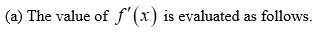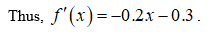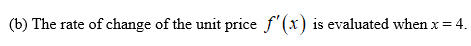# The demand function for the Luminar desk lamp is given byp = f(x) = −0.1x2 − 0.3x + 32where x is the quantity demanded in thousands and p is the unit price in dollars.(a)Find f '(x). (b)What is the rate of change of the unit price (in dollars per 1,000 lamps) when the quantity demanded is 4,000 units (x = 4)?\$   per 1,000 lampsWhat is the unit price (in dollars) at that level of demand?

Question
27 views
The demand function for the Luminar desk lamp is given by
p = f(x) = −0.1x2 − 0.3x + 32
where x is the quantity demanded in thousands and p is the unit price in dollars.
(a)
Find
f '(x).

(b)
What is the rate of change of the unit price (in dollars per 1,000 lamps) when the quantity demanded is 4,000 units (x = 4)?
\$   per 1,000 lamps
What is the unit price (in dollars) at that level of demand?
check_circle

Step 1

Consider the given demand function for the Luminar desk lamp,

Step 2Step 3...

### Want to see the full answer?

See Solution

#### Want to see this answer and more?

Solutions are written by subject experts who are available 24/7. Questions are typically answered within 1 hour.*

See Solution
*Response times may vary by subject and question.
Tagged in

### Derivative